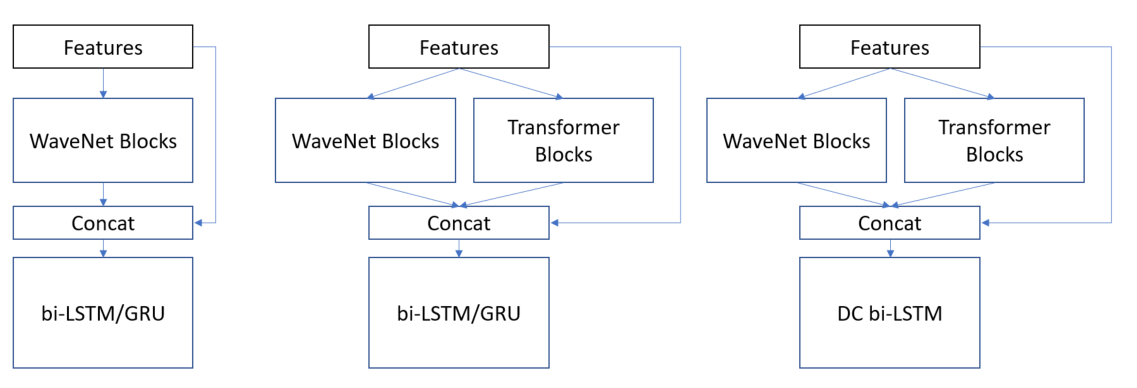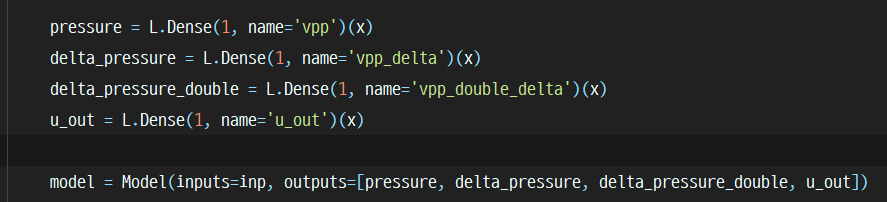Nov 03, 20213 min read ☕ (Last updated: Jan 19, 2023)

# (Kaggle) Ventilator Pressure Prediction - 20th (top 1%) place solution

## TL;DR

Our solutions are focused on the two parts.

1. Deep learning architectures
2. multi-task learning that enables us to train the model more stable

## Pre-Processing

We didn't take much effort into developing pre-processing. The pre-processing publicly available is good enough for us!

So, move on to the next chapter : )

## Model

### Arhcitecture

We developed a total of 3 types of architecture.

1. WaveNet-wise blocks + bi-LSTM/GRU
2. WaveNet-wise blocks + Transformer Encoder + bi-LSTM/GRU
3. WaveNet-wise blocks + Transformer Encoder + Densely-Connected bi-LSTMWe encode the features with CNN & Transformer blocks, then take them into the bi-LSTM layers. The second model (WaveNet-wise blocks + Transformer Encoder + bi-LSTM/GRU) achieved the best CV score among our models.

Actually, we can't submit those models as a single model because development & training were finished with the last few days of the challenge left. However, To assume with CV score (there're usually 0.02 gap between CV & LB scores in our cases), scores are like below.

Here's the CV/LB table. Actually, we can't submit those models as a single model because development & training were finished with the last few days of the challenge left. However, To assume with CV score (there're usually 0.02 gap between CV & LB scores in our cases), maybe we can guess the LB score : )

• w/o pseudo label, 15 folds (stratified), w/o post-process, same seed.
model CV LB
WaveNet-wise blocks + bi-LSTM/GRU 0.13914 0.1186
WaveNet-wise blocks + Transformer Encoder + bi-LSTM/GRU 0.136130 0.1160 (assume)
WaveNet-wise blocks + Transformer Encoder + Densely-Connected bi-LSTM 0.137117 0.1170 (assume)
• w/ pseudo label, 15 folds (stratified), w/o post-process, same seed.
model cv lb
WaveNet + bi-LSTM/GRU 0.110575 0.1147
WaveNet + TRFM + bi-LSTM/GRU 0.107606 ?
WaveNet + TRFM + DC bi-LSTM 0.110126 ?

% There're 3 variants of models based on WaveNet + bi-LSTM/GRU. Posted one is the baseline version. % there's validation set mismatch between w/o and w/ pseudo label experiment.

I'll also attach this table into the post!

In the early stage of the competition, I found that using delta pressure (diff of pressure) as an auxiliary loss raises CV/LB scores (+0.01 ~ 0.015 boosts).

Similarly, delta of delta pressure (double diff pressure) as an auxiliary loss also boosts the performance by about +0.002 ~ 3 on CV/LB scores.

df['delta_pressure'] = (df['pressure'] - df.groupby('breath_id')['pressure'].shift(1)).fillna(0).values
df['delta_delta_pressure'] = (df['delta_pressure'] - df.groupby('breath_id')['delta_pressure'].shift(1)).fillna(0).values

In the TF 2.x code, we use like below.## Post-Processing / Ensemble

We can't find any huge boosts from the post-processing. However, Ensemble methods do bring an improvement!

First, We tuned an ensemble weight with Optuna library based on the OOF predictions. After that, calculating gap of predictions (spread) and taking mean (w/o min, max predictions) or median selectively by spread threshold (optimized based on OOF) on the whole models (include the weighted ensemble prediction). It improves +0.0001 ~ CV boost compared with just taking a median.

Ultimately, We ensembled the 5 models based on the above architectures with some differences (e.g. layers, features). Got CV 0.096600 LB 0.1131 PB 0.1171 (low CV score is due to training on the pseudo label)

## Summary

### Works

• cross-validation
• stratified k fold (on R, C factors) is better than group k fold
• 15 folds is better than 10 folds (about +0.002 CV/LB boosts)
• pseudo label
• single model : CV 0.13914 LB 0.1186 PB 0.1226 -> CV 0.11057 LB 0.1147 PB 0.1186 (about LB/PB +0.004 improvement)
• only for u_out == 0 works better
• delta pressure (about +0.01 ~ 0.015 CV/LB boosts)
• delta of delta pressure (about +0.002 ~ 0.004 CV/LB boosts)